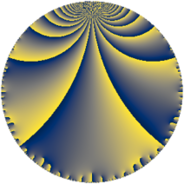Properties

 Label 441.6.yLevel $441$ Weight $6$ Character orbit 441.y Rep. character $\chi_{441}(25,\cdot)$ Character field $\Q(\zeta_{21})$ Dimension $3336$ Sturm bound $336$

Related objects

Defining parameters

 Level: $$N$$ $$=$$ $$441 = 3^{2} \cdot 7^{2}$$ Weight: $$k$$ $$=$$ $$6$$ Character orbit: $$[\chi]$$ $$=$$ 441.y (of order $$21$$ and degree $$12$$) Character conductor: $$\operatorname{cond}(\chi)$$ $$=$$ $$441$$ Character field: $$\Q(\zeta_{21})$$ Sturm bound: $$336$$

Dimensions

The following table gives the dimensions of various subspaces of $$M_{6}(441, [\chi])$$.

Total New Old
Modular forms 3384 3384 0
Cusp forms 3336 3336 0
Eisenstein series 48 48 0

Trace form

 $$3336 q - 5 q^{2} - 13 q^{3} - 8837 q^{4} - 108 q^{5} - 178 q^{6} - 35 q^{7} + 44 q^{8} - 963 q^{9} + O(q^{10})$$ $$3336 q - 5 q^{2} - 13 q^{3} - 8837 q^{4} - 108 q^{5} - 178 q^{6} - 35 q^{7} + 44 q^{8} - 963 q^{9} + 38 q^{10} - 8 q^{11} - 6094 q^{12} - 186 q^{13} + 3112 q^{14} + 776 q^{15} - 139205 q^{16} - 2071 q^{17} - 2875 q^{18} + 50 q^{19} - 2789 q^{20} - 861 q^{21} + 59 q^{22} - 437 q^{23} + 10732 q^{24} + 168742 q^{25} - 4826 q^{26} + 33719 q^{27} - 824 q^{28} - 77615 q^{29} - 4614 q^{30} + 2156 q^{31} + 6119 q^{32} + 6965 q^{33} - 37 q^{34} + 24328 q^{35} - 68110 q^{36} - 33553 q^{37} - 22486 q^{38} + 2749 q^{39} - 1021 q^{40} - 46637 q^{41} + 21646 q^{42} - 9249 q^{43} - 7129 q^{44} + 79767 q^{45} + 123278 q^{46} + 135485 q^{47} + 111601 q^{48} - 4295 q^{49} + 9759 q^{50} + 32166 q^{51} + 152696 q^{52} - 283342 q^{53} + 111765 q^{54} + 77660 q^{55} - 514211 q^{56} + 31473 q^{57} - 65152 q^{58} + 186773 q^{59} + 17233 q^{60} + 77120 q^{61} + 301464 q^{62} + 136173 q^{63} - 2160596 q^{64} + 23209 q^{65} + 490530 q^{66} - 1258 q^{67} + 638317 q^{68} + 78550 q^{69} + 60026 q^{70} - 137431 q^{71} - 158650 q^{72} + 41988 q^{73} - 131868 q^{74} + 661446 q^{75} + 17339 q^{76} - 20363 q^{77} + 693915 q^{78} + 46958 q^{79} + 1063915 q^{80} + 599005 q^{81} - 58 q^{82} + 1139827 q^{83} - 229691 q^{84} - 3130 q^{85} + 22386 q^{86} - 712931 q^{87} - 30664 q^{88} - 123440 q^{89} - 547758 q^{90} + 21423 q^{91} - 387307 q^{92} - 1138049 q^{93} + 192731 q^{94} + 517 q^{95} + 747420 q^{96} + 34667 q^{97} + 1364595 q^{98} + 1035014 q^{99} + O(q^{100})$$

Decomposition of $$S_{6}^{\mathrm{new}}(441, [\chi])$$ into newform subspaces

The newforms in this space have not yet been added to the LMFDB.